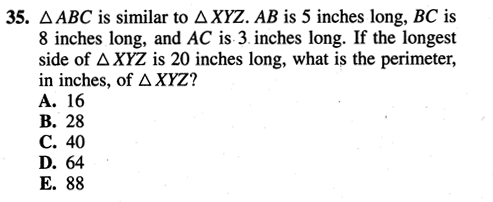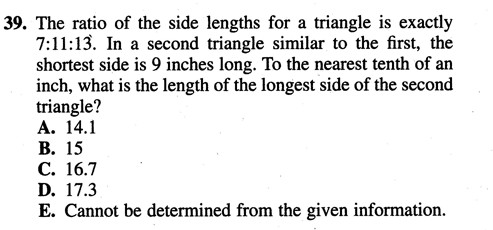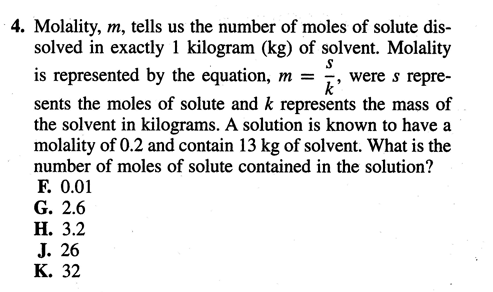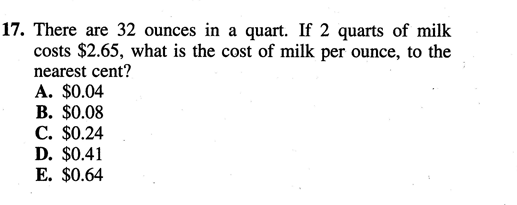Imagination is more important than knowledge... Albert Einstein

Guess is more important than calculation --- Knowhowacademy.com

Ratio - Algebraic Ratio

For ACT
Number of Total Problems: 28.
FOR PRINT ::: (Book)

 Problem Num : 1 Type: None Section:Ratio Theme:None Adjustment# : Difficulty: 1Category Algebraic Ratio
Analysis

 Problem Num : 2 Type: None Section:Ratio Theme:None Adjustment# : Difficulty: 1Category Algebraic Ratio
Analysis

 Problem Num : 3 Type: None Section:Ratio Theme:None Adjustment# : Difficulty: 1Category Algebraic Ratio
Analysis

 Problem Num : 4 Type: None Section:Ratio Theme:None Adjustment# : Difficulty: 1Category Algebraic Ratio
Analysis

 Problem Num : 5 Type: None Section:Ratio Theme:None Adjustment# : Difficulty: 1Category Algebraic Ratio
Analysis

 Problem Num : 6 Type: None Section:Ratio Theme:None Adjustment# : Difficulty: 1Category Algebraic Ratio
Analysis

 Problem Num : 7 Type: None Section:Ratio Theme:None Adjustment# : Difficulty: 1Category Algebraic Ratio
Analysis

 Problem Num : 8 Type: None Section:Ratio Theme:None Adjustment# : Difficulty: 1Category Algebraic Ratio
Analysis

 Problem Num : 9 Type: None Section:Ratio Theme:None Adjustment# : Difficulty: 1Category Algebraic Ratio
Analysis

 Problem Num : 10 Type: None Section:Ratio Theme:None Adjustment# : Difficulty: 1Category Algebraic Ratio
Analysis